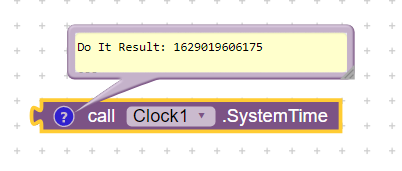# Firebase and Primary key

Hello everyone,
How to create a unique id in Firebase database ? I tried to implement it but I was unable to.
Any help would be appreciatedMaybe you could use timestamp as unigue idOr do what firebase do:
(this is javascript)

``````function getUID() {

for(var newID,success=!1;!success;)20==(newID=generatePushID()).length&&(newID,success=!0);

return newID;

}

generatePushID=function(){

var r="0123456789ABCDEFGHIJKLMNOPQRSTUVWXYZabcdefghijklmnopqrstuvwxyz",e=0,t=[];

return function(){var o=(new Date).getTime(),n=o===e;e=o;for(var a=new Array(8),h=7;h>=0;h--)a[h]=r.charAt(o%64),o=Math.floor(o/64);

if(0!==o)throw new Error("convert entire timestamp");

var f=a.join("");if(n){for(h=11;h>=0&&63===t[h];h--)t[h]=0;t[h]++}else for(h=0;h<12;h++)t[h]=Math.floor(64*Math.random());

for(h=0;h<12;h++)f+=r.charAt(t[h]);

return f}}();
``````
1 Like